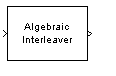Main Content

# Algebraic Interleaver

Reorder input symbols using algebraically derived permutation table

## Library

Block sublibrary of Interleaving

•## Description

The Algebraic Interleaver block rearranges the elements of its input vector using a permutation that is algebraically derived. The Number of elements parameter, N, indicates how many numbers are in the input vector. This block accepts a column vector input signal.

The block accepts the following data types: `int8`, `uint8`, `int16`, `uint16`, `int32`, `uint32`, `boolean`, `single`, `double`, and fixed-point. The output signal inherits its data type from the input signal.

The Type parameter indicates the algebraic method that the block uses to generate the appropriate permutation table. Choices are `Takeshita-Costello` and `Welch-Costas`. Each of these methods has parameters and restrictions that are specific to it:

• If you set Type to `Welch-Costas`, then N + 1 must be prime. The Primitive element parameter is an integer, A, between 1 and N that represents a primitive element of the finite field GF(N + 1). This means that every nonzero element of GF(N + 1) can be expressed as A raised to some integer power.

In a Welch-Costas interleaver, the permutation maps the integer k to mod(Ak,N + 1) - 1.

• If you set Type to `Takeshita-Costello`, then N must be 2m for some integer m. The Multiplicative factor parameter, k, must be an odd integer less than N. The Cyclic shift parameter, h, must be a nonnegative integer less than N.

A Takeshita-Costello interleaver uses a length-N cycle vector whose nth element is

`$c\left(n\right)=\mathrm{mod}\left(k\cdot \frac{n\cdot \left(n-1\right)}{2},N\right)+1,\text{\hspace{0.17em}}n$`

for integers n between 1 and N. The intermediate permutation function is obtained by using the following relationship:

`$\Pi \left(c\left(n\right)\right)=c\left(n+1\right)$`

where

`$n=1:N$`

The interleaver's actual permutation vector is the result of cyclically shifting the elements of the permutation vector, π, by the Cyclic shift parameter, h.

## Parameters

Type

The type of permutation table that the block uses for interleaving.

Number of elements

The number of elements, N, in the input vector.

Multiplicative factor

The factor used to compute the interleaver's cycle vector. This field appears only if Type is set to `Takeshita-Costello`.

Cyclic shift

The amount by which the block shifts indices when creating the permutation table. This field appears only if Type is set to `Takeshita-Costello`.

Primitive element

An element of order N in the finite field GF(N+1). This field appears only if Type is set to `Welch-Costas`.

## Pair Block

Algebraic Deinterleaver

## References

 Heegard, Chris and Stephen B. Wicker. Turbo Coding. Boston: Kluwer Academic Publishers, 1999.

 Takeshita, O. Y. and D. J. Costello, Jr. "New Classes Of Algebraic Interleavers for Turbo-Codes." Proc. 1998 IEEE International Symposium on Information Theory, Boston, Aug. 16-21, 1998. 419.

## See Also

### Blocks

Introduced before R2006a

## Support

#### Bridging Wireless Communications Design and Testing with MATLAB

Download white paper